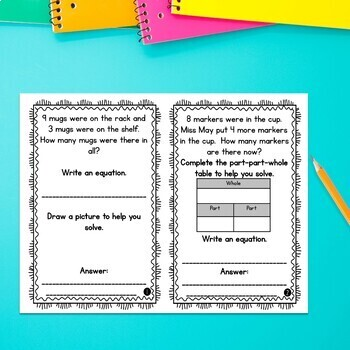# 1st Grade Word Problem of the Day Story Problems | Addition & Subtraction1st
Subjects
Standards
Resource Type
Formats Included
• Zip
Pages
288 pages
\$21.00
Bundle
List Price:
\$27.00
You Save:
\$6.00
\$21.00
Bundle
List Price:
\$27.00
You Save:
\$6.00

### Description

These 1st Grade Story Problems of the Day contain real-world applications of addition and subtraction in the first grade math standards. Students build problem solving skills through guided and independent practice of the standards daily.

This nine month word problems bundle includes 32 story problems for each month of the school year (Sept-May). That's 288 word problems!

Skills included:

• addition and subtraction within 10

• addition and subtraction within 20 with unknowns in all positions

• using money (dollar amounts and with only pennies)

• adding three numbers within 20

• compare word problems

• part-part-whole word problems

• start-change-result word problems

• writing equations

• completing part-part-whole tables

• completing start-change-end tables

• creating tables

• drawing a picture to represent the problem

• writing answers in complete sentences

• addition and subtraction within 100 without regrouping

Each month includes two files: each problem printed twice per page in half sheets, or problems printed two per page in half sheets. 32 pages are provided each month so that a page can be completed each day, if you wish, with additional pages going home for independent practice. These can be easily printed and stapled for daily use, or these are also perfect for inclusion in interactive notebooks.

The 1st Grade Word Problems of the Day:

Sept | Oct | Nov | Dec | Jan | Feb | March | April | May

You can also check out the Back to School Freebie.

Looking for word problem type practice? Check out my Addition & Subtraction within 20 Digital Word Problems

CLICK HERE to follow me and be notified of future products as soon as they are posted.

_______________________________________________________________

You may not redistribute, edit, sell, or otherwise post this product on the internet. You may, however, post a link for others topurchase themselves.

Total Pages
288 pages
N/A
Teaching Duration
1 Year
Report this Resource to TpT
Reported resources will be reviewed by our team. Report this resource to let us know if this resource violates TpT’s content guidelines.

### Standards

to see state-specific standards (only available in the US).
Use addition and subtraction within 20 to solve word problems involving situations of adding to, taking from, putting together, taking apart, and comparing, with unknowns in all positions, e.g., by using objects, drawings, and equations with a symbol for the unknown number to represent the problem.
Solve word problems that call for addition of three whole numbers whose sum is less than or equal to 20, e.g., by using objects, drawings, and equations with a symbol for the unknown number to represent the problem.
Apply properties of operations as strategies to add and subtract. If 8 + 3 = 11 is known, then 3 + 8 = 11 is also known. (Commutative property of addition.) To add 2 + 6 + 4, the second two numbers can be added to make a ten, so 2 + 6 + 4 = 2 + 10 = 12. (Associative property of addition.)
Understand subtraction as an unknown-addend problem. For example, subtract 10 – 8 by finding the number that makes 10 when added to 8.
Relate counting to addition and subtraction (e.g., by counting on 2 to add 2).